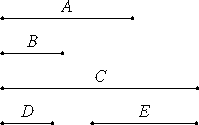# Proposition 7

If a composite number multiplied by any number makes some number, then the product is solid.

Let the composite number A multiplied by any number B make C.

I say that C is solid.VII.Def.13

Since A is composite, it is measured by some number D. Let there be as many units in E as times that D measures A

Since D measures A according to the units in E, therefore E multiplied by D makes A. And, since A multiplied by B makes C, and A is the product of D and E, therefore the product of D and E multiplied by B makes C.

Therefore C is solid, and D, E, and B are its sides.

Therefore, if a composite number multiplied by any number makes some number, then the product is solid.

Q.E.D.

## Guide

Numbers with at least two factors are plain numbers; those with at least three are solid numbers.